Why do we need it. What is it. Types of activation functions.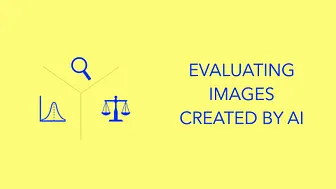What's the problem. What to measure. What metrics are available.What are the categories. What tools are available. How do they compare to each other.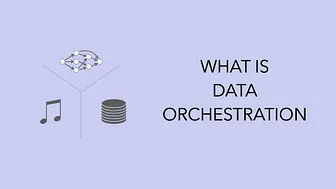What is it. Why do we need it. List of tools available.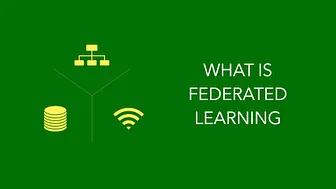Why do we need it. How does it work. Where is it used.Why do we need it. How does it work. Where is it used.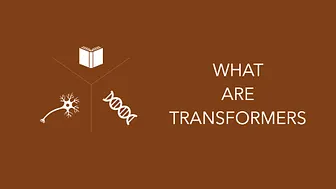What are they. Why do we need them. Where are they used.Why do we need it. How does it work. Where is it used.What is it. Why do we need it. What makes it special.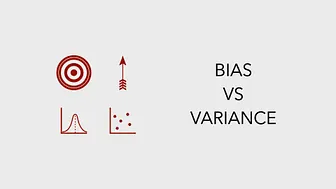Why look at bias and variance. Errors resulting from them. Bias vs variance tradeoff.What exactly is it. Why is it relevant. Where is it used.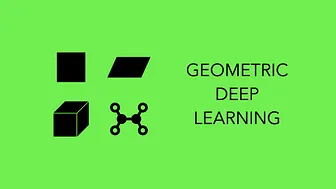What it's all about. Why is it important. How it's used in the real world.